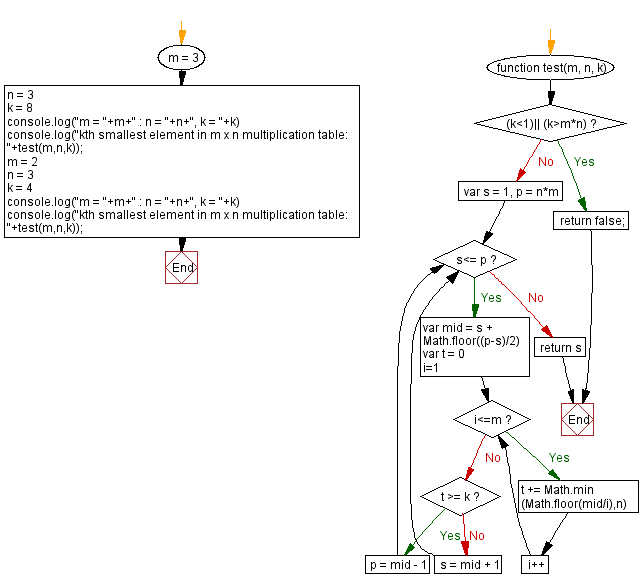# JavaScript: Kth smallest number in a multiplication table

## JavaScript Math: Exercise-111 with Solution

Write a JavaScript program that creates a multiplication table of size m x n using integers where 1 <= k <= m * n. Return the kth smallest element in the said multiplication table.

In mathematics, a multiplication table is a mathematical table used to define a multiplication operation for an algebraic system.
The decimal multiplication table was traditionally taught as an essential part of elementary arithmetic around the world, as it lays the foundation for arithmetic operations with base-ten numbers. Many educators believe it is necessary to memorize the table up to 9 x 9.
The illustration below shows a table up to 12 x 12, which is a size commonly used nowadays in English-world schools.

 x 0 1 2 3 4 5 6 7 8 9 10 11 12 0 0 0 0 0 0 0 0 0 0 0 0 0 0 1 0 1 2 3 4 5 6 7 8 9 10 11 12 2 0 2 4 6 8 10 12 14 16 18 20 22 24 3 0 3 6 9 12 15 18 21 24 27 30 33 36 4 0 4 8 12 16 20 24 28 32 36 40 44 48 5 0 5 10 15 20 25 30 35 40 45 50 55 60 6 0 6 12 18 24 30 36 42 48 54 60 66 72 7 0 7 14 21 28 35 42 49 56 63 70 77 84 8 0 8 16 24 32 40 48 56 64 72 80 88 96 9 0 9 18 27 36 45 54 63 72 81 90 99 108 10 0 10 20 30 40 50 60 70 80 90 100 110 120 11 0 11 22 33 44 55 66 77 88 99 110 121 132 12 0 12 24 36 48 60 72 84 96 108 120 132 144

Test Data:
(3,3,8) -> 6
(2,3,4) -> 3

Sample Solution:

HTML Code:

``````<!DOCTYPE html>
<html>
<meta charset="utf-8">
<title>JavaScript program to Kth smallest number in a multiplication table</title>
<body>

</body>
</html>
```
```

JavaScript Code:

``````function test(m, n, k) {
if ((k<1)|| (k>m*n))
return false;
var s = 1, p = n*m
while (s<= p) {
var mid = s + Math.floor((p-s)/2)

var t = 0
for (i=1;i<=m;i++) {
t += Math.min(Math.floor(mid/i),n)
}

if (t >= k) {
p = mid - 1
} else {
s = mid + 1
}
}
return s
}

m = 3
n = 3
k = 8
console.log("m = "+m+" : n = "+n+", k = "+k)
console.log("kth smallest element in m x n multiplication table: "+test(m,n,k));
m = 2
n = 3
k = 4
console.log("m = "+m+" : n = "+n+", k = "+k)
console.log("kth smallest element in m x n multiplication table: "+test(m,n,k));
```
```

Sample Output:

```m = 3 : n = 3, k = 8
kth smallest element in m x n multiplication table: 6
m = 2 : n = 3, k = 4
kth smallest element in m x n multiplication table: 3
```

Flowchart:Live Demo:

See the Pen javascript-math-exercise-111 by w3resource (@w3resource) on CodePen.

Improve this sample solution and post your code through Disqus

What is the difficulty level of this exercise?

Test your Programming skills with w3resource's quiz.

﻿